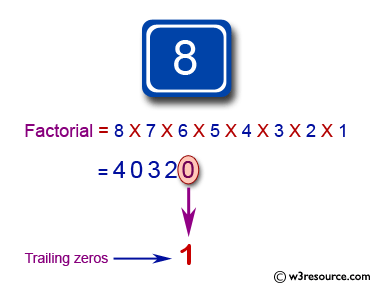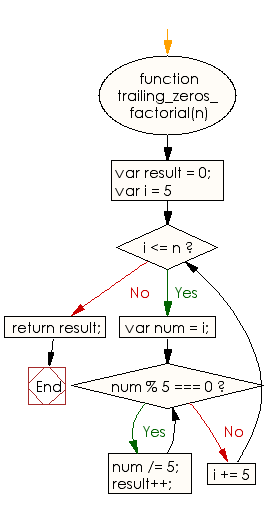# JavaScript: Find the number of trailing zeros in the decimal representation of the factorial of a given number

## JavaScript Basic: Exercise-112 with Solution

Write a JavaScript program to find the number of trailing zeros in the decimal representation of the factorial of a given number.

Pictorial Presentation:Sample Solution:

HTML Code:

``````<!DOCTYPE html>
<html>
<meta charset="utf-8">
<meta name="viewport" content="width=device-width">
<title> Find the number of trailing zeros in the decimal representation of the factorial of a given number</title>
<body>

</body>
</html>
```
```

JavaScript Code:

``````function trailing_zeros_factorial(n) {
var result = 0;
for (var i = 5; i <= n; i += 5) {
var num = i;
while (num % 5 === 0) {
num /= 5;
result++;
}
}
return result;
}

console.log(trailing_zeros_factorial(8))
console.log(trailing_zeros_factorial(9))
console.log(trailing_zeros_factorial(10))
``````

Sample Output:

```1
1
2
```

Flowchart:ES6 Version:

``````function trailing_zeros_factorial(n) {
let result = 0;
for (let i = 5; i <= n; i += 5) {
let num = i;
while (num % 5 === 0) {
num /= 5;
result++;
}
}
return result;
}

console.log(trailing_zeros_factorial(8))
console.log(trailing_zeros_factorial(9))
console.log(trailing_zeros_factorial(10))
``````

Live Demo:

See the Pen javascript-basic-exercise-112 by w3resource (@w3resource) on CodePen.

What is the difficulty level of this exercise?

Test your Programming skills with w3resource's quiz.

﻿

## JavaScript: Tips of the Day

Returns the sum of the powers of all the numbers from start to end (both inclusive)

Example:

```const sumPower = (end, power = 2, start = 1) =>
Array(end + 1 - start)
.fill(0)
.map((x, i) => (i + start) ** power)
.reduce((a, b) => a + b, 0);
console.log(sumPower(10)); // 385
console.log(sumPower(10, 3)); // 3025
console.log(sumPower(10, 3, 5)); // 2925
```

Output:

```385
3025
2925
```